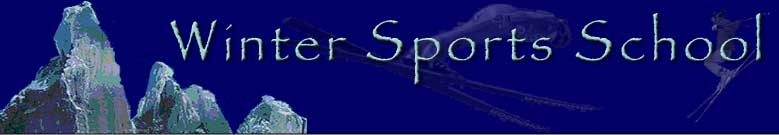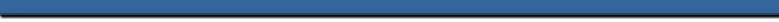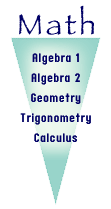The purpose of the mathematics program at the Winter Sports School is to develop an understanding of skills and concepts that will enable the student to gain a better appreciation and understanding for logical procedure, and the knowledge to enrich their comprehension of the language of mathematics. In order for these goals to be met, we offer the students a wide assortment of opportunities in both the practical application of mathematics and the pure theoretical exploration of the language.Alternate Regular Advanced Accelerated Pre-Algebra Algebra I Algebra I Algebra I Algebra I Algebra II Algebra II Algebra II Algebra II Geometry Geometry Geometry Geometry Trigonometry Trigonometry Trigonometry Calculus A Calculus A Calculus B

 We deal with world-class athletes at the Winter Sports School. These students have needs that are not found in more traditional settings. We find that some of these athletes will invariably be absent due to training or competitions. Instructors have developed many ways to ensure that these students are able to continue with their educations while they are away. Sometimes students will be given assignments in advance or they will make up the work upon their return. Instructors can stay in touch with the student via e-mail, Internet, and fax answering questions and presenting ideas. Instructors may take the time to meet with the student on an individual basis for private tutoring sessions prior to or post competition. We know that the athletes that come to the Winter Sports School are in pursuit of an education that can be achieved in coordination with their rigorous training and competition schedules. Through the teaching of basic skills and concepts, the mathematics curriculum strives to respond to the prior experience, current knowledge, aspirations, and learning styles of each individual student.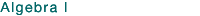This course is designed for students who have successfully completed pre-algebra. The course is designed to prepare students for Algebra II. It will create an understanding of how to apply mathematics and algebra. Topics to be covered will include translating written statements to equations, proportional reasoning, using number lines, solving linear equations and inequalities, graphing functions and an introduction to polynomials. Graphing calculators are recommended but not required. Text: Algebra I by Larson, Boswell, Kanold, & Stiff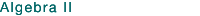Prerequisite: Algebra I. This course presents a more in-depth study of the topics included in Algebra I. The students will study functions and relations, the development of formulas and their applications, conic sections, matrices, etc. Graphing calculators will be used as a means to enhance lesson topics. The students will engage in hands-on activities to demonstrate the actual mathematical applications of the topics under investigation. Graphing calculators are required (TI-82 or similar) Text: Algebra II by Larson, Boswell, Kanold, & StiffPrerequisite: Algebra II. This course presents a rigorous in-depth study of Euclidean and non-Euclidean geometry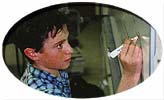with an introduction to trigonometry. Emphasis will be placed on inductive and deductive reasoning. The students will deal with the real world applications of geometry. Text: Geometry by Boyd, Burril, Cummins, Kanold, & Molloy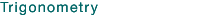This course presents the study of right triangles and the use of the six basic functions to solve practical mathematical problems. The students will focus on the topics of graphs and the transformation of the parent functions, solving trigonometric equations and using identities to prove a variety of trigonometric statements. Graphing calculators are required (TI-82 or similar). Text: Trigonometry-Functions and Applications by Paul A. Foerster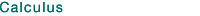This course is the study of mathematics of the continuum. Both the theory of elementary functions and real world applications of mathematics will be studied. The students will learn differential and integral calculus and their applications. Graphing calculators are required (TI-82 or similar). Text: Calculus of a Single Variable by Larson, Hostetler, & Edwards- Copyright © 2002; Winter Sports School, Park City, UT. All Rights Reserved -